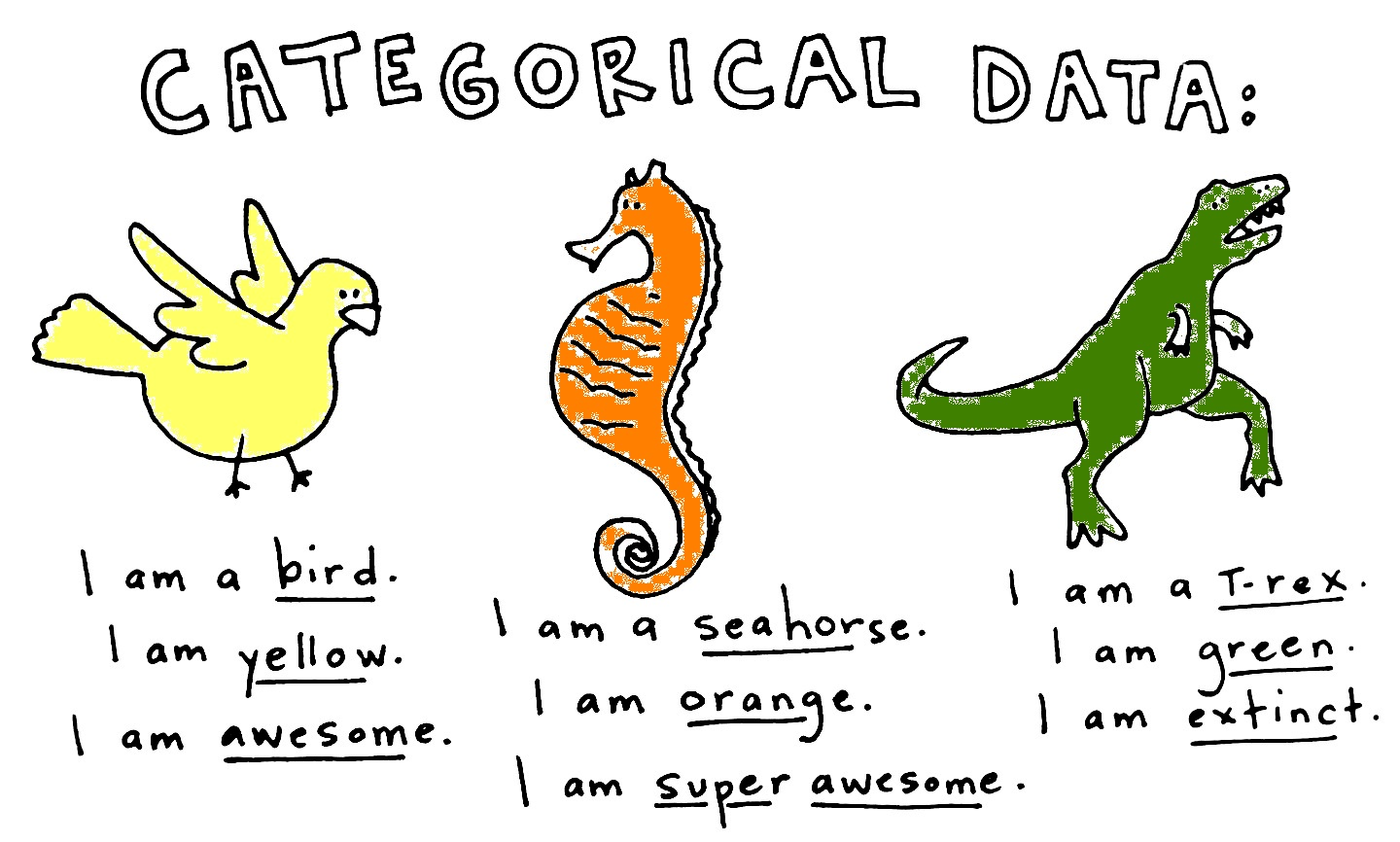## Categorical Data

Data that falls into a discrete number of categories.

The different possible values of a categorical variable are called levels

bird_sightings <-
factor(c("pigeon", "pigeon", "goshawk",
"eagle", "goshawk"))
levels(bird_sightings)
##  "eagle"   "goshawk" "pigeon"

## Example - Species Occurrences

myData <- data.frame(
species = sample(c("Homo_sapiens","Bison_bison", "Canis_latrans"),1000, replace=T)
)
head(myData)
##      site       species
## 2 forest1  Homo_sapiens
## 3 forest1   Bison_bison
## 5 forest1 Canis_latrans
## 6 forest1   Bison_bison

## Example - Species Occurrences

R has useful tools for counting occurrences

table(myData$site,myData$species)
##
##           Bison_bison Canis_latrans Homo_sapiens
##   forest1         148           182          169
##   meadow2         162           163          176

This is called a contingency table. Analysis of categorical data always operates on contingency tables.

## Contingency Tables

A more real (but still fake) example.

Site A. afarensis Ar. ramidus Aepyceros melampus
Aramis 0 90 220

Made up of counts or frequencies of observations in each category.

Rows are indexed by $$i$$ and columns are indexed by $$j$$.

There are $$n$$ rows in a table and $$m$$ columns.

Analyzing contingency tables requires the raw counts: not percentages, proportions, etc.

## Hypothesis Testing

Site A. afarensis Ar. ramidus Aepyceros melampus
Aramis 0 90 220

Null hypothesis: no association between $$site$$ variable and the $$species$$ variable.

Alternative hypothesis: There is a relationship between the $$site$$ variable and the $$species$$ variable

To reject the null hypothesis, we need to ask, what are the expected values of the cells, assuming no association?

## Hypothesis Testing - Expected Values

Site A. afarensis Ar. ramidus Aepyceros melampus
Aramis 0 90 220

Intuitively, what would you expect the value of each cell to be assuming the row and column variable are unrelated???

## Hypothesis Testing - Expected Values

Going back to probability, the probability of being an A. afarensis at Hadar is a shared event made up of two simple events:

• being A. afarensis

We simply multiply these probabilities and multiply by the sample size

## Hypothesis Testing - Expected Values

• Probablility of being A. afarensis
• $$120 / 1030 = 0.1165$$
• Probablility of occuring at Hadar
• $$720 / 1030 = 0.6990$$
• Probablility of being A. afarensis at Hadar
• $$0.6990 * 0.1165 = 0.0814$$

Expected value of this cell is $$0.0814 * 1030 = 83.84$$

## Hypothesis Testing - Expected Values

Shortcut for computing expected cell frequencies:

$\hat{Y}_{i,j} = \frac{row\ total\times{column\ total}}{sample\ size} = \frac{\sum\limits_{j=1}^mY_{i,j}\times\sum\limits_{i=1}^nY_{i,j}}{N}$

Volunteer: calculate by hand on board!

Site A. afarensis Ar. ramidus Aepyceros melampus
Aramis 0 90 220

## Hypothesis Testing - Chi-Square

Karl Pearson came up with a test statistic to quantify how much the observed counts differ from the expected values:

$X^2_{Pearson} = \sum\limits_{all\ cells}\frac{(Observed-Expected)^2}{Expected}$

This is analogous to the residual sum of squares in linear modeling.

## Hypothesis Testing - Chi-Square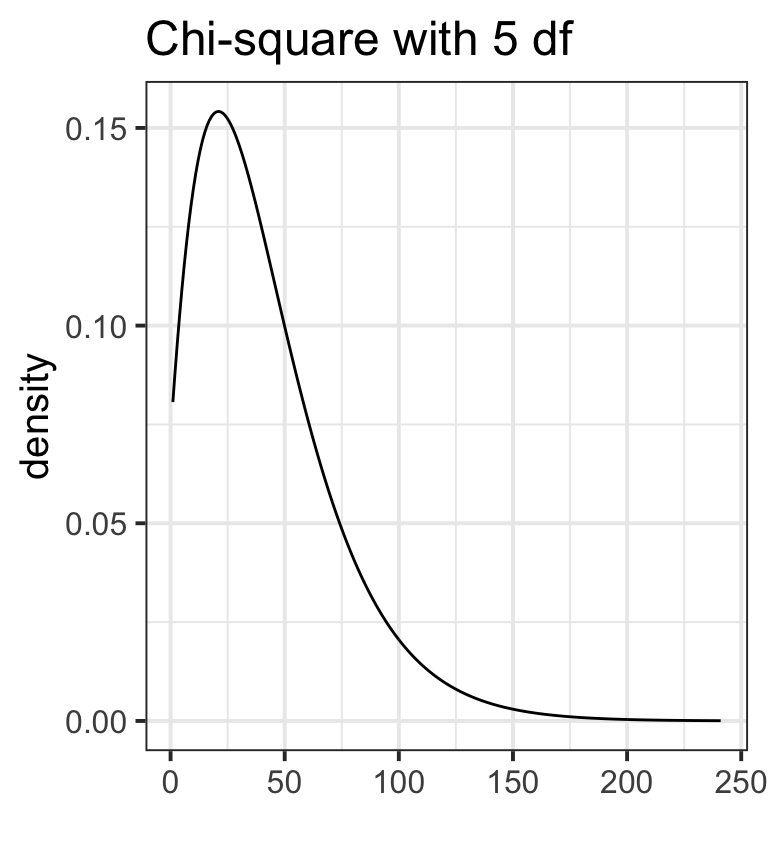Chi-square has a known parametric distribution

Can be used to calculate p-values

## Chi-square in R

myTable <- table(myData$site,myData$species)
chisq.test(myTable)
##
##  Pearson's Chi-squared test
##
## data:  myTable
## X-squared = 1.8167, df = 2, p-value = 0.4032

Note, you can either pass two vectors of data, or a pre-made contingency table.

## Fisher's Exact Test

More appropriate when sample sizes are low.

General rule is to use Fisher's if expected value for any cell is < 5.

fisher.test(myTable)
##
##  Fisher's Exact Test for Count Data
##
## data:  myTable
## p-value = 0.4066
## alternative hypothesis: two.sided

## Goodness of Fit Tests

These test how closely observed data fit some underlying distribution (e.g., binomial, uniform, normal)

For discrete cases, chi-square can be used as a goodness of fit statistic.

## Chi-square goodness of fit

For instance: say we counted the frequency of a A. afarensis in 4 different geological strata through time.

afarensis <- c(24, 32, 19, 36)

## Chi-square goodness of fit

We can use chi-square to test how well this fits a uniform distribution:

chisq.test(afarensis)
##
##  Chi-squared test for given probabilities
##
## data:  afarensis
## X-squared = 6.3694, df = 3, p-value = 0.09496

## Chi-square goodness of fit

We could specify some other distribution by passing a vector of probabilities.

chisq.test(afarensis, p=c(.4, .1, .1, .4))
##
##  Chi-squared test for given probabilities
##
## data:  afarensis
## X-squared = 55.937, df = 3, p-value = 4.333e-12

## Continuous Goodness of Fit - KS

The Kolmogorov-Smirnov is a commonly used goodness of fit test for continuous data.

The KS test compares the cumulative distribution function (CDF) of a set of observed data to a theoretical distribution.

## KS-Test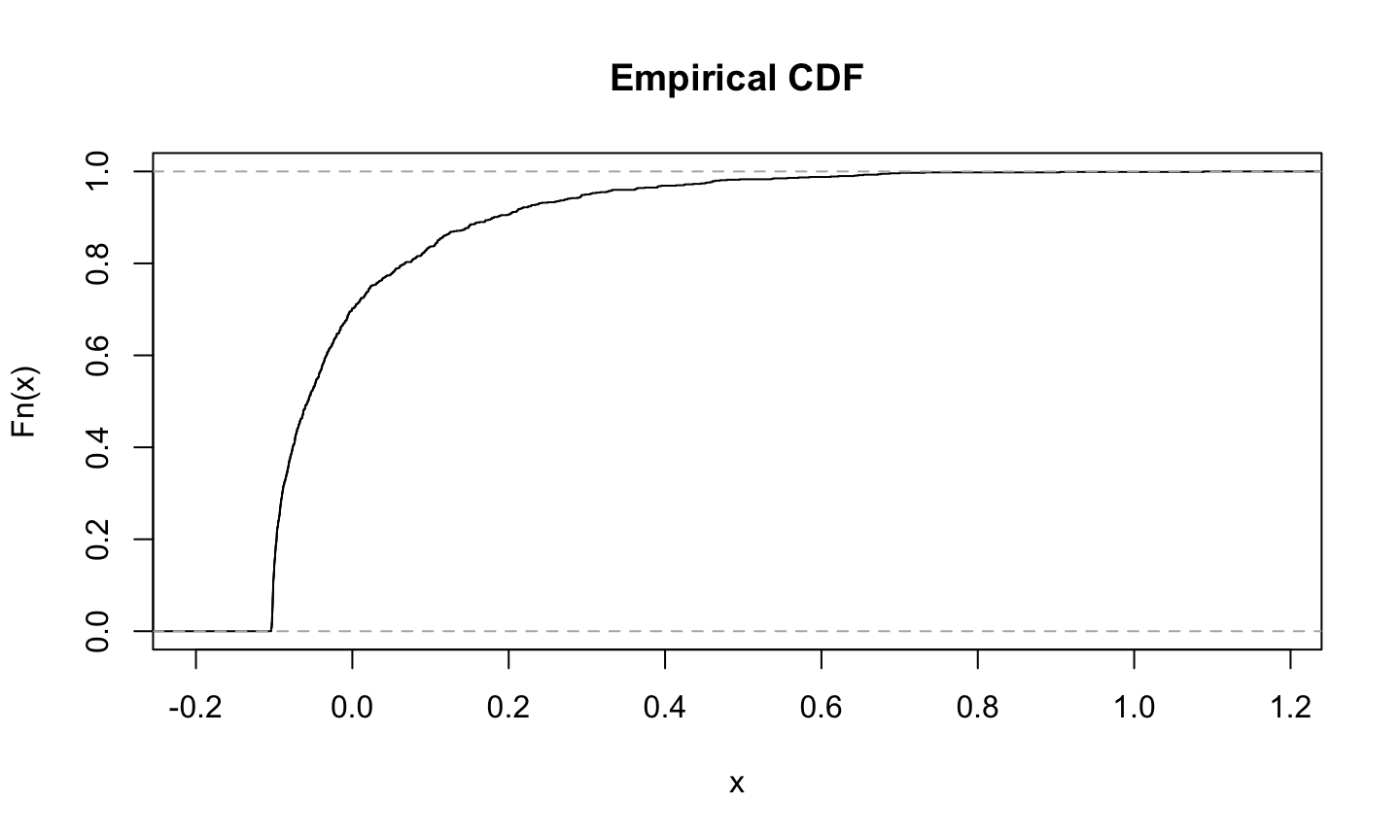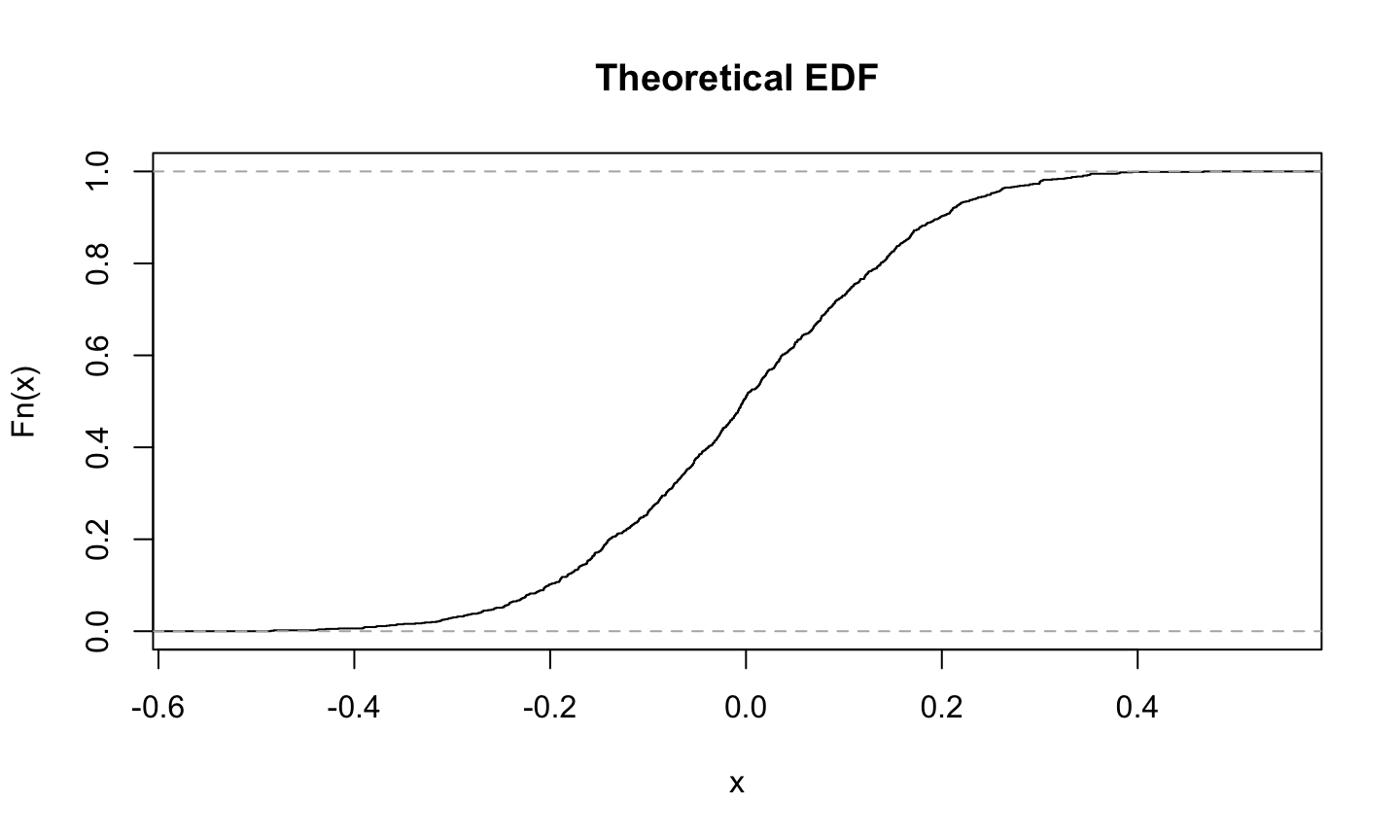## KS-Test

The single largest deviation of the empirical from the theoretical is the KS statistic. This is used to compute a p-value.

Can be used for any distribution, not just the normal distribution.

## KS-Test in R

ks.test(rnorm(100), "pnorm")
##
##  One-sample Kolmogorov-Smirnov test
##
## data:  rnorm(100)
## D = 0.060459, p-value = 0.8582
## alternative hypothesis: two-sided

## KS-Test in R

ks.test(rnorm(100)^2, "pnorm")
##
##  One-sample Kolmogorov-Smirnov test
##
## data:  rnorm(100)^2
## D = 0.50022, p-value < 2.2e-16
## alternative hypothesis: two-sided

## Challenge

Read in this dataset on political party affiliation and gender in the USA. http://hompal-stats.wabarr.com/datasets/party_affiliation.txt

Make a plot to visualize the data that looks like the following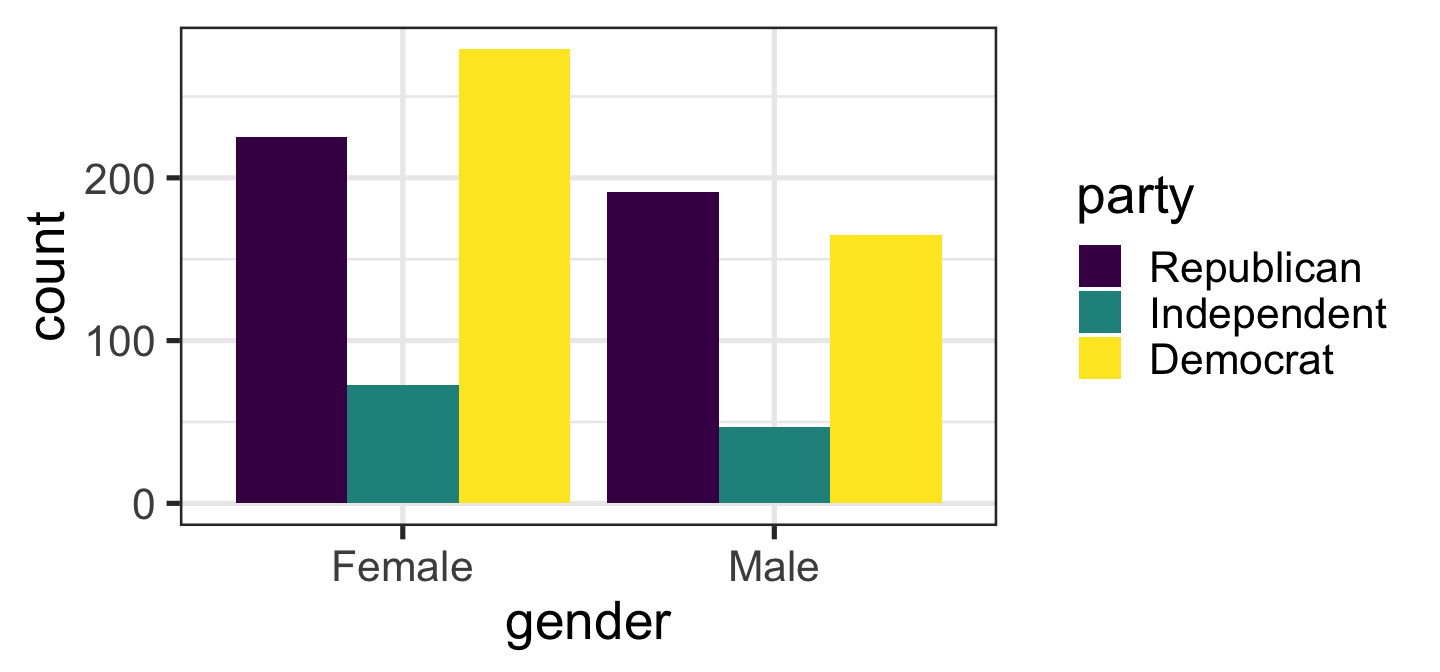## Challenge

Use chi-square to test the hypothesis that there is a gendered difference in party affiliation.

## Challenge

Check out the built-in dataset trees.

Are the tree heights drawn from a normal distribution? Test this hypothesis using the appropriate goodness of fit test.